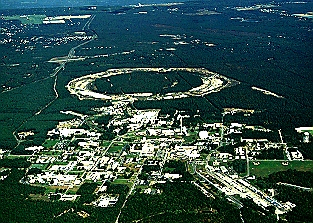$$\require{cancel}$$

# 3.3: Main Experimental Facilities

•• Contributed by Niels Walet
• Professor (Physics) at University of Manchester

Let me first list a couple of facilities with there energies, and then discuss the facilities one-by-one.

Table $$\PageIndex{1}$$: Fixed target facilities, and their beam energies
accelerator facility particle energy
KEK Tokyo $$p$$ 12 GeV
SLAC Stanford $$e^-$$ 25GeV
PS CERN $$p$$ 28 GeV
AGS BNL $$p$$ 32 GeV
SPS CERN $$p$$ 250 GeV
Tevatron II FNL $$p$$ 1000 GeV
Table $$\PageIndex{2}$$: Colliding beam facilities, and their beam energies
accelerator facility particle & energy (in GeV)
CESR Cornell $$e^+(6)+e^-(6)$$
PEP Stanford $$e^+(15)+e^-(15)$$
Tristan KEK $$e^+(32)+e^-(32)$$
SLC Stanford $$e^+(50)+e^-(50)$$
LEP CERN $$e^+(60)+e^-(60)$$
SppS CERN $$p(450)+\bar{p}(450)$$
Tevatron I FNL $$p(1000)+\bar{p}(1000)$$
LHC CERN $$e^-(50)+p(8000)$$
$$p(8000)+\bar{p}(8000)$$

# SLAC (B factory, Babar)

Stanford Linear Accelerator Center, located just south of San Francisco, is the longest linear accelerator in the world. It accelerates electrons and positrons down its 2-mile length to various targets, rings and detectors at its end. The PEP ring shown is being rebuilt for the B factory, which will study some of the mysteries of antimatter using B mesons. Related physics will be done at Cornell with CESR and in Japan with KEK.Figure $$\PageIndex{1}$$: A picture of SLAC

# Fermilab (D0 and CDF)

Fermi National Accelerator Laboratory, a high-energy physics laboratory, named after particle physicist pioneer Enrico Fermi, is located 30 miles west of Chicago. It is the home of the world’s most powerful particle accelerator, the Tevatron, which was used to discover the top quark.Figure $$\PageIndex{2}$$: A picture of Fermilab

# CERN (LEP and LHC)

CERN (European Laboratory for Particle Physics) is an international laboratory where the W and Z bosons were discovered. CERN is the birthplace of the World-Wide Web. The Large Hadron Collider (see below) will search for Higgs bosons and other new fundamental particles and forces.Figure $$\PageIndex{3}$$: A picture of CERN

# Brookhaven (RHIC)

Brookhaven National Laboratory (BNL) is located on Long Island, New York. Charm quark was discovered there, simultaneously with SLAC. The main ring (RHIC) is 0.6 km in radius.Figure $$\PageIndex{4}$$: A picture of Brookhaven National Lab

# Cornell (CESR)

The Cornell Electron-Positron Storage Ring (CESR) is an electron-positron collider with a circumference of 768 meters, located 12 meters below the ground at Cornell University campus. It is capable of producing collisions between electrons and their anti-particles, positrons, with centre-of-mass energies between 9 and 12 GeV. The products of these collisions are studied with a detection apparatus, called the CLEO detector.Figure $$\PageIndex{5}$$: A picture of the Cornell accelerator

# DESY (Hera and Petra)

The DESY laboratory, located in Hamburg, Germany, discovered the gluon at the PETRA accelerator. DESY consists of two accelerators: HERA and PETRA. These accelerators collide electrons and protons.Figure $$\PageIndex{6}$$: A picture of HERA

# KEK (tristan)

The KEK laboratory, in Japan, was originally established for the purpose of promoting experimental studies on elementary particles. A 12 GeV proton synchrotron was constructed as the first major facility. Since its commissioning in 1976, the proton synchrotron played an important role in boosting experimental activities in Japan and thus laid the foundation of the next step of KEK’s high energy physics program, a 30 GeV electron-positron colliding-beam accelerator called TRISTAN.Figure $$\PageIndex{7}$$: A picture of KEK

# IHEP

Institute for High-Energy Physics, in the People’s Republic of China, performs detailed studies of the tau lepton and charm quark.Figure $$\PageIndex{8}$$: A picture of IHEP